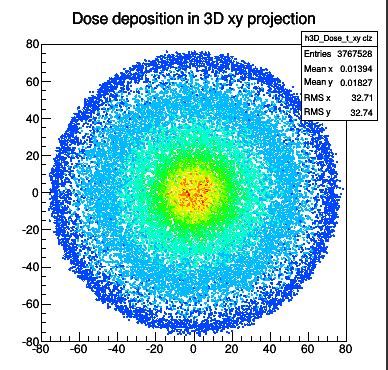# TH2D contour lines

Hi,

I was trying to make contour plots similar to what is in the pdf attached. This is where I got stuck, please see the output attached.

``````name_file="./Dosimetry_Detector_tot.root";
TFile * f = new TFile(name_file,"r");
h3_tot=(TH3D*)f->Get("h3D_Dose_t");
h2_sum_z=(TH2D*)h3_tot->Project3D("xy colz");

h2_sum_z->SetContour(10);
h2_sum_z->DrawClone("Cont1");``````

This draws an output as the attached picture. This should be 10 equidistant contours, well I don’t know how this compares to percentage. If this matches up with percentages then I would like to draw a line around them and mark with a number as in the pdf.

If not then I need to find a way to define the contours by percentages then draw lines around and numbers.

Any suggestions would be appreciated.

Thank you,
Tibor

The root file:
https://www.dropbox.com/s/3a6llsjqt6woxx5/Dosimetry_Detector_tot.root?dl=0
Isodose wisconsin results.pdf (1.32 MB)It looks like the colored background is obtained with the option COL and the contour with CONT3. I wrote the following macro to simulate that:

``````{
TCanvas *c1 = new TCanvas();
gStyle->SetOptStat(0);
TH2F *h1 = new TH2F("h1","h1",50,-4,4,50,-4,4);
Double_t a,b;
for (Int_t i=0;i<306500;i++) {gRandom->Rannor(a,b);h1->Fill(a-1.5,b-1.5);}

for (Int_t i=0;i<550;i++) {h1->Fill(3.5,3);}
for (Int_t i=0;i<450;i++) {h1->Fill(3.5,2);}
for (Int_t i=0;i<350;i++) {h1->Fill(3.5,1);}
for (Int_t i=0;i<250;i++) {h1->Fill(3.5,0);}
for (Int_t i=0;i<150;i++) {h1->Fill(3.5,-1);}
for (Int_t i=0;i< 50;i++) {h1->Fill(3.5,-2);}
for (Int_t i=0;i<  5;i++) {h1->Fill(3.5,-3);}

double contours;
contours = 3;
contours = 100;
contours = 900;
contours = 1500;

h1->DrawCopy("colz");
h1->SetContour(4,contours);
h1->Draw("cont3 same");
h1->SetLineColor(kRed);
}
``````

Thank you, I’ve tried something like this before and cant get lines out of them. Please see the output attached.

``````{
gStyle->SetOptStat(0);

name_file="./Dosimetry_Detector_tot.root";
TFile * f = new TFile(name_file,"r");
h3_tot=(TH3D*)f->Get("h3D_Dose_t");

TH2D* h3xy;
h3xy=(TH2D*)h3_tot->Project3D("xy");

h3xy->DrawCopy("colz");
h3xy->SetContour(4);
h3xy->Draw("cont3 same");
h3xy->SetLineColor(kRed);
``````

It is because your histogram has many bins varying quickly from one bin to its neighbors.
The color plot looks smooth because the bins are small and, even if the changes have a very high variation frequency, the overall long distance variation in slow. But the contour plot is making its way around all the noise…

May be you can try to smooth the data used to draw the contour or try to have less bins for the contour.

Thank you! It looks better after smooth()

``````  gStyle->SetOptStat(0);

name_file="./Dosimetry_Detector_tot.root";
TFile * f = new TFile(name_file,"r");
h3_tot=(TH3D*)f->Get("h3D_Dose_t");

TH2D* h3xy;
h3xy=(TH2D*)h3_tot->Project3D("xy");

h3xy->Smooth();
h3xy->DrawCopy("colz");
h3xy->SetContour(4);
h3xy->Draw("cont3 same");
h3xy->SetLineColor(kRed);
``````

I’m trying to do the same thing with pyroot. For the line

double contours;

how can I put it in pyroot? Thank you.

Hi @1116,

Try using Python’s `array`:
https://docs.python.org/3/library/array.html

Cheers,

Enric

Hi,

I’ve got the following error after trying to input it as an array:

TypeError: void TH1::SetContour(Int_t nlevels, const Double_t* levels = 0) =>
could not convert argument 2

Hi,

This works for me:

``````>>> import ROOT
>>> h1 = ROOT.TH2F("h1","h1",50,-4,4,50,-4,4)
>>> h1.Fill(3.5,3)
2335
>>> from array import array
>>> x = array( 'd' )
>>> x.append(3.0)
>>> x.append(100)
>>> x.append(900)
>>> x.append(1500)
>>> h1.SetContour(4,x)
``````

Thank you, it works pretty well!